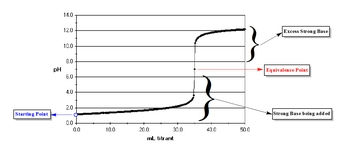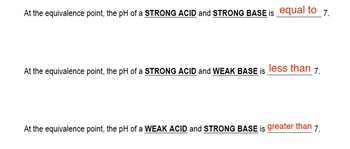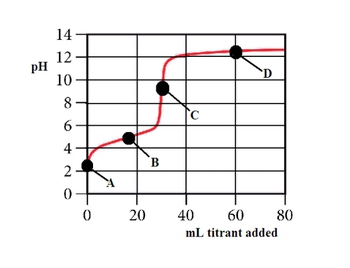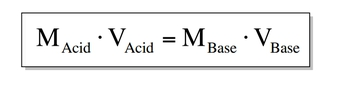## General Chemistry

Learn the toughest concepts covered in Chemistry with step-by-step video tutorials and practice problems by world-class tutors

18. Aqueous Equilibrium

# Acid and Base Titration Curves

Titration Curves

Titration curves help us to understand the neutralization occurring between an acid and base in order to identify the equivalence point.

Equivalence Point

The equivalence point is when the moles of acid and base titrating are equal in amount.

1
concept

## Understanding an Acid–Base Titration Curve4m
Play a video:

In a typical titration we begin with an acid or base and slowly add either a Strong Acid or Strong Base.2
concept

## The Equivalence Point1m
Play a video:
we've already answered this first part, we're gonna say at the equivalence point, the ph of a strong acid and strong base is equal 27. Now, if we're looking at a strong acid and a weak base, just remember whoever is weak will dictate what kind of solution you have at the end. Since we're dealing with a strong acid, the acid is stronger now than the base. So it's gonna say, since I'm stronger, I'm gonna want my solution at the end to be acidic. So anytime you're tight trading a weak acid, a strong base together at the equivalence point, the ph is going to be less than seven, it's gonna be acidic and it's acidic because the acid is stronger. Whoever is stronger, will tell us what kind of solution we have in the first example, They both were strong. So we wind up with a draw which means neutral. That's why the ph is equal to seven. Now, for the last scenario we have a weak acid and a strong base. Now the base is stronger. So it's going to dictate what kind of solution we have. Because we're dealing with a strong base, it's gonna want to have a basic solution. So the solution has to have a ph greater than seven at the equivalence point. So, just remember these fundamentals and as we start to do more acid and based filtration questions, keep this in mind as we face every type of question we're going to see. So just remember the equivalence point is when we have equal moles of acid and base. And depending on what we're tight trading, whether they both are strong or one is strong or weak, the equivalence point could have a ph equal to seven, less than seven or greater than seven.

Depending on the types of acids and bases mixing, at the equivalence point, the pH can be less than, greater than or equal to 7.3
example

## The following questions refer to the titration curve given below.1) The titration curve shows the titration of    a) a strong acid with a strong base    b) a weak acid with a strong base    c) a strong base with a strong acid    d) a weak base with a strong acid      2) Which point on the titration curve represents a region where a buffer solution has formed6m
Play a video:
Hey everyone. So here it says the following questions refer to the hydration curve given by law. So if we take a look at this situation curve we have, let's see on our Y axis. We have our ph volumes from 0 to 14 on our X axis, we have our milliliters of tightrope being added. We have points A, B, C and D. So there's quite a few things we can talk about in terms of this. But let's look at the questions and we'll see um what gets at it or what gets labeled. Alright, so here the titillation curve shows the tight rations of what so different combinations. If we look here, we need to locate what the equivalence point will be. Remember, the equivalence point can be determined by looking at what volume do we have? The sharpest rise within our ph we can see that happens around 30 mls where our sharpest rising peach happens here, midway through there is our equivalence points or equivalence point here is point C. And if we trace over here, we can see that point C has a ph of around nine or so, maybe a little bit higher than nine, but it's about nine. So think about what combination Between acid and base. Give me a page that's greater than seven at the equivalence point. So here, remember that happens when we have a weak acid being titrate, ID by a strong base. So that's the combination, weak acid strong base. That will give me a ph above seven for the equivalence point. So here we want a weak acid with a strong base would be the second option here, which point of the hydration curve represents a region where a buffer solution has formed. Remember a buffer exists between these two Before we get to the equivalence point. But remember for buffer to exist in some way to be generated, some of the titrate has to have been added at point A There is no tie trend that's been added yet, so there is no buffer that's been formed. All we have initially is weak acid. When we get to a point B. This represents our half equivalence point. This represents our ideal buffer. Remember at the half equivalent points where we have equal amounts of weak acid and its conjugate base. This is where we have our most ideal buffer. So, point B represents where a buffer has been formed, which point on the tire traction curve represents the equivalence point. We already discussed this, we said that was point C. And then finally, which of the following would be the best indicator to use in the tight rations. Remember an indicator can approximate what your equivalence point is by using the endpoint. That's the ph in which the indicator changes colors. So here we have our equivalence point around nine maybe slightly higher than nine. So we want to use an indicator that has uh basically a p K. That's close to that value. So, if we look here, we see that our last option here has a PK equal to nine, it's p K is going to be very close to the ph of the equivalence point. So that means it's testing for the endpoint and that endpoints around nine which is right next to the equivalence point, ph So this would be the best indicator to use. So again, the best indicator to use for a PhD attrition curve is the indicator that has a p k a closest to the equivalent point. Ph. So again, in this case, it will be our last option, which has a PK A equal to nine.An indicator represents a weak acid or base in low concentration that changes color in an acid base titration. An indicator shows us the location of our endpoint, which is located near our equivalence point.

4
example

## The acid form of an indicator is red and its anion is blue3m
Play a video:
Hey, guys, In this new video, we're gonna continue with our discussion of Ph tight Trish in curves when looking at indicators. So in this first example, it says the acid form of an indicator And what we should realize here is that an indicator is really just a weak acid. So an indicator has an acidic h on it. So the acid form is gonna be h A. And it's an ion form Means it's removed. It's h so not a minus. So the acid form of an indicator is red and it's an ion form. Its conjugate base form is blue. The value for this indicator is 10 to the negative nine. What will be the approximate ph range over which this indicator changes color. Now, what you should realize here is that in order to figure out the pH range oven indicator, all you have to remember is this The pH range of an indicator equals PK plus or minus one. So we have the K. So how do we find a peek? A. If we have k, just take the negative log of it. So we're gonna say p k equals negative log of K a so equals negative. Log off 10 to the negative nine. So the P K equals nine. And we just said that the PH range for an indicator is PK a plus or minus one. So the ph range is nine plus or minus one, which means nine minus one is eight to nine, plus one is 10. So the range would be between eight and 10. And that's what we have to remember Now. I'm gonna give you guys a hint on the next question, so just understand it like this. We're going to say we have a peek A that is equal to nine. Well, we should realize here, if your P H is equal to nine, we have pH and PK equally each other. And what did we say about that? We said that when pH equals P k, you're at the half equivalents points, so you have equal amounts off your acid form and your base form. So at this moment, h A equals a minus. And we're gonna say here at P K nine, that means that the Ph has to be at least nine for us to start to remove the acidic H off of our indicator. So what color would our indicator be below Ph of nine? What color would our indicator be above ah ph of nine. This will help us understand the next practice question. So remember this pattern attempt to answer this question on your own and then don't worry, Come back. Look at the next following video. You'll see an example of me explaining how to approach this problem and what the correct answer is. Good luck, guys.

An indicator, like a buffer, has an optimal range in which it works most effectively:

pka +/- 1.

5
Problem

What will be the color of the indicator in the above question in a solution that has a pH of 6?

6
example

## Consider the titration of 100.0 mL of 0.016 M H2SO4 with 0.400 M NaOH at the equivalence point6m
Play a video:

If at the equivalence point, the moles of acid equal the moles of base and moles equal molarity multiplied by liters then at the equivalence point we can use the formula:7
example

## Consider the titration of 40.0 mL of 0.0800 M HCl with 0.0160 M Ca(NH2)2.a) How many milliliters of 0.0160 M Ca(NH2)2 are required to reach the equivalence point2m
Play a video:

When dealing with the equivalence point make sure you correctly calculate the molarity for both the acid and base.

8
example

## Consider the titration of 40.0 mL of 0.0800 M HCl with 0.0160 M Ca(NH2)2.b) What is the pH of this solution1m
Play a video: